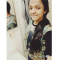Class Central is learner-supported. When you buy through links on our site, we may earn an affiliate commission.

# Mathematical Methods for Quantitative Finance

This course may be unavailable.

### Overview

##### Class Central Tips
The Mathematical Methods for Quantitative Finance course reviews the mathematical methods fundamental for the study of quantitative and computational finance. The areas of focus include calculus and multivariable calculus, constrained and unconstrained optimization, and linear algebra.

Topics covered include the following:

• Functions and inverse functions
• Limits, derivatives, partial derivatives, and chain rule
• Integrals and multiple integrals, changing the order of differentiation and integration
• Taylor series approximations
• Newton’s method
• Lagrange multiplier method
• Vector and matrix arithmetic, determinants, eigenvalue-eigenvector decomposition, singular value decomposition
• Numerical methods for optimization
Course goal:
Upon completion of the course students will know the fundamental mathematical concepts needed to effectively study quantitative finance areas such as fixed income, options and derivatives, portfolio optimization, and quantitative risk management.

Course Objectives
:

Upon completion of the course students will:

1. Understand the concept of a limit, differentiation, and integration;
2. Be able to compute partial derivatives and multiple integrals;
3. Understand the utility of matrix decompositions;
4. Be able to use Lagrange multipliers to solve constrained optimization problems; and
5. Apply the above methods to problems arising in finance.

Kjell Konis

## Reviews

4.6 rating, based on 7 Class Central reviews

Start your review of Mathematical Methods for Quantitative Finance

•Drishti Bansal
• Derrick Thompson
• John Walsh

### Never Stop Learning.

Get personalized course recommendations, track subjects and courses with reminders, and more.